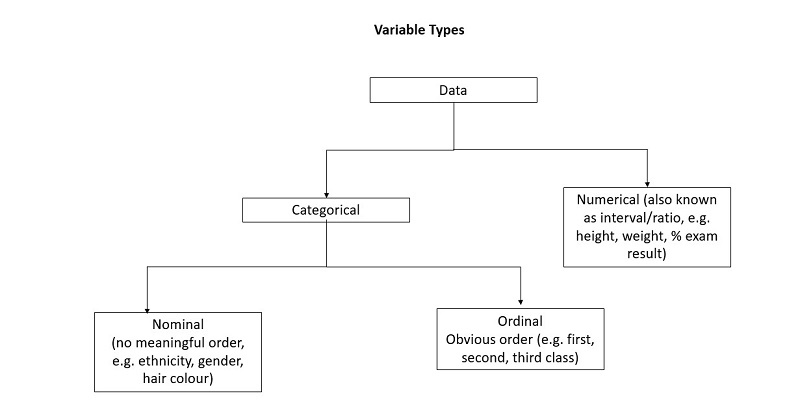# Statistical tests

## Statistical test terminology

Choosing the right statistical test to use with your data can be difficult.

You need to understand some basic statistical terms to understand which statistical test is the most appropriate for your data. These terms include:

• numerical and categorical (nominal and ordinal) data types
• dependent (outcome) and independent (predictor or explanatory) variables
• independent or repeated measures data
• parametric and non-parametric testing.

### Numerical and categorical data types

Data can be classified as either categorical or numerical.

Examples of numerical data include variables such as height, weight, marks in an exam. In statistics, different words may have a similar meaning so the term numerical is sometimes referred to as interval or ratio.

Within the categorical data type, there are two sub-groups:

• Nominal variable: this data has no meaningful order, eg ethnicity, gender, hair colour
• Ordinal variable: this data has an obvious order, eg first, second, thirdData variable types split into categorical (with sub divisions of nominal and ordinal) and numerical

### Dependent and independent variables

Variables are also described as dependent and independent. A dependent variable may sometimes also be known as a outcome variable. An independent variable may also be known as a predictor variable.

A dependent variable is one that depends on other variable(s). For example someone’s exam result (dependent) might depend on the number of hours they study (independent).

### Independent or repeated measures data

If you have repeated samples of data taken over time (eg pulse rate before and after exercise; water quality sample at the same point in a river taken during the morning, afternoon and evening) this is called repeated measures, paired or within-subjects.

If you have samples of data from independent groups (eg pulse rates of children and adults) this is called independent measures, or unpaired or between-subjects.

### Parametric and non-parametric tests

Parametric tests are the most common type of tests and make certain assumptions about the parameters of a population. For example, parametric tests assume that the sample has been randomly selected from the representative population and that the distribution of the data in the population has a known underlying distribution, commonly the normal distribution.

Parametric tests require the numerical data type whereas non-parametric tests require categorical data that are nominal or ordinal.